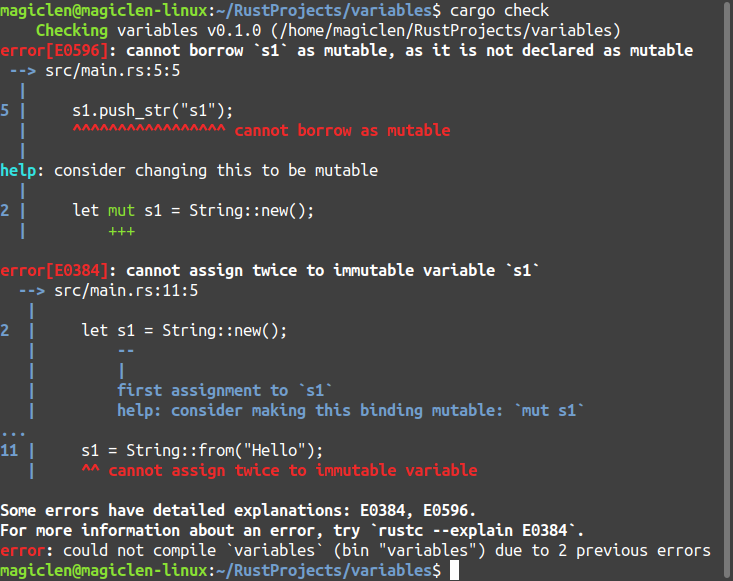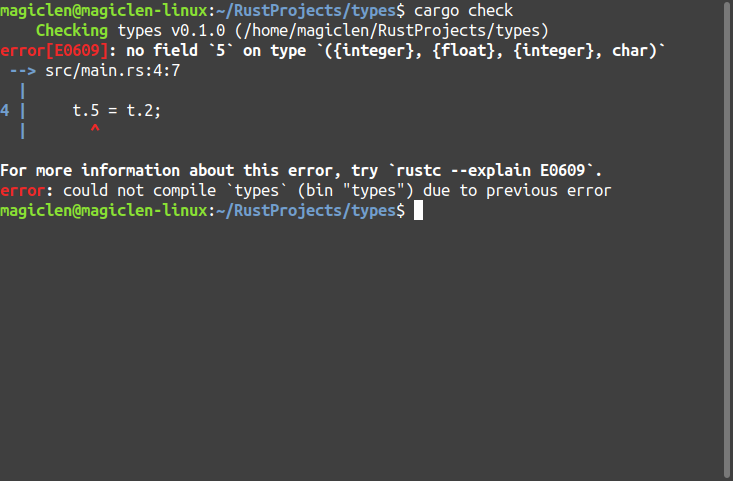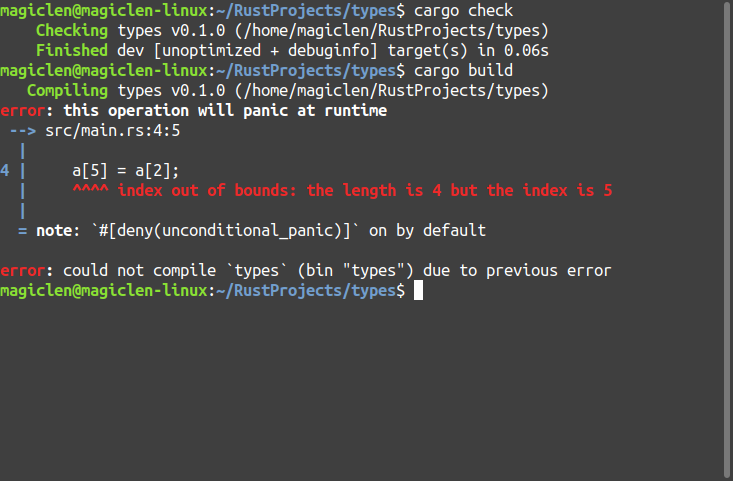### 變數

```fn main() {
let s1 = String::new();
let mut s2 = String::new();
s1.push_str("s1");
s2.push_str("s2");
println!("{}", s1);
println!("{}", s2);
s1 = String::from("Hello");
s2 = String::from("Hi");
println!("{}", s1);
println!("{}", s2);
}```「不可變的變數」不就跟常數(constant)是一樣嗎？雖然它們看起來都是值不可以改變的名稱，但還是有一些差異。在Rust程式語言中，提供了「const」關鍵字來宣告常數，與用「let」宣告變數不同的是，「const」不允許加上「mut」關鍵字來讓常數變成可變的，因為「可變的常數」就根本不是常數了嘛！再來就是「const」宣告常數時，必須要明確地給出常數的型別，因為常數的值在Rust程式的編譯階段時，就需要確定下來了，換句話說，常數也無法儲存程式執行階段才能得到的資料。常數可以被宣告在任何的scope，包含全域的scope，且名稱習慣都使用大寫字母，並使用底線區隔不同的單字。例如：

`const MAX_POINTS: u32 = 100000;`

```fn main() {
let x = 5;

let x = x + 1;

let x = x * 2;

println!("The value of x is: {}", x);
}```

The value of x is: 12

```fn main() {
let spaces = "   ";

let spaces = spaces.len();
}```

```fn main() {
let mut spaces = "   ";

spaces = spaces.len();
}```

### 資料型別

Rust是「靜態型別」(Static Typing)的程式語言，在編譯階段就要完全決定好變數的型別，因此可以讓Rust程式在編譯階段就知道要怎麼樣儲存和處理該類型的資料。使用「let」關鍵字宣告變數的時候，通常可以不用事先指定好該變數的型別，因為編譯器會自動根據第一次指派給變數的值來推論變數的型別。舉例來說：

```fn main() {
let a;
let b = 1;
a = 2;
}```

`let guess: i32 = guess.trim().parse().expect("Please type a number!");`

#### 純量型別(Scalar Types)

##### 整數

in: -2n-1 ~ 2n-1-1

un: 0 ~ 2n-1

`let x = 12_345;`

```let h = 0xff;
let o = 0o377;
let b = 0o11111111;```

```fn main() {
let c0 = b'0';
let cA = b'A';
println!("0 = {}, A = {}", c0, cA);
}```

0 = 48, A = 65

```fn main() {
let a = 1i8; // i8
let a: i8 = 1; // i8
let a = 1 as i8; // i8
let a = 1i16; // i16
let a: i16 = 1; // i16
let a = 1 as i16; // i16
let a = 1; // i32
// let a = 2147483648; // i32
let a = 2147483648i64; // i64
let a: i64 = 2147483648; // i64
let a = 2147483648 as i64; // i64
// let a = 9223372036854775808; // i32
let a = 9223372036854775808i128; // i128
let a: i128 = 9223372036854775808; // i128
let a = 9223372036854775808 as i128; // i128

let a = 1u8; // u8
let a: u8 = 1; // u8
let a = 1 as u8; // u8
let a = b'1'; // u8
let a = 1u16; // u16
let a: u16 = 1; // u16
let a = 1 as u16; // u16
let a = 1u32; // u32
let a: u32 = 1; // u32
let a = 1 as u32; // u32
let a = 1u64; // u64
let a: u64 = 1; // u64
let a = 1 as u64; // u64
let a = 1u128; // u128
let a: u128 = 1; // u128
let a = 1 as u128; // u128
}```

##### 浮點數

```fn main() {
let a = 1.0f32; // f32
let a = 1f32; // f32
let a: f32 = 1.0; // f32
let a = 1.0 as f32; // f32
let a = 1 as f32; // f32

let a = 1f64;
let a = 1.0; // f64
let a = 1 as f64; // f64
}```

```let a: i32 = 1.0;
let a: f64 = 1;```

```let a = 1.0 as i32;
let a = 1 as f64;```

##### 字元

`let c = 'C';`

「char」型別可以表示「Unicode Scalar」編碼的值，也就是說，除了ASCII字元外，還可以表示中文、日文、韓文(CJK)的文字，也能表示表情符號。

#### 複合型別(Compound Types)

##### 數組

`let t = (500, 6.4, 1, 'A'); // (i32, f64, i32, char)`

`let t: (i32, f64, i32, char) = (500, 6.4, 1, 'A');`

```let t = (500, 6.4, 1, 'A');
let (a, b, c, d) = t; // a: i32, b: f64, c: i32, d: char```

```let t = (500, 6.4, 1, 'A');
let a;
let b;
let c;
let d;
(a, b, c, d) = t;```

```let t = (500, 6.4, 1, 'A');
let a;
let b;
let c;
let d;
a = t.0;
b = t.1;
c = t.2;
d = t.3;```

```let mut t = (500, 6.4, 1, 'A');
t.0 = t.2;```

```fn main() {
let mut t = (500, 6.4, 1, 'A');
t.0 = t.2;
}```##### 陣列

`let a = [2, 4, 6, 8, 10]; // [i32; 5]`

`let a: [i32; 5] = [2, 4, 6, 8, 10];`

```#![feature(slice_patterns)]

fn main() {
let a = [2, 4, 6, 8, 10];
let [v, w, x, y, z] = a;
}```

```let a = [2, 4, 6, 8, 10];
let v;
let w;
let x;
let y;
let z;
[v, w, x, y, z] = a;```

```let a = [2, 4, 6, 8, 10];
let v;
let w;
let x;
let y;
let z;
v = a;
w = a;
x = a;
y = a;
z = a;```

```let mut a = [1, 2, 3, 4];
a = a;```

```let mut a = [1, 2, 3, 4];
let x = a.get(0);```

```fn main() {
let mut a = [1, 2, 3, 4];
a = a;
}````[每個欄位的初始元素值; 陣列長度]`

```fn main() {
let mut a = [0; 4];
a = 1;
a = 2;
a = 3;
a = 4;
}```

### 函數

```fn 函數名稱(參數名稱和型別) {
主體的程式敘述
}```

```fn another_function(name: &str) {
println!("Hi, {}.", name);
}```

Rust程式語言並未限制定義函數的順序。舉例來說，以下兩個程式都是可以編譯的：

```fn main() {
another_function("world");
}

fn another_function(name: &str) {
println!("Hi, {}.", name);
}```
```fn another_function(name: &str) {
println!("Hi, {}.", name);
}

fn main() {
another_function("world");
}```

```fn main() {
let x = {
let y = 10;
let z = 4;
println!("y = {}, z = {}", y, z);
y + z
};
println!("x = {}", x);
}```

```fn 函數名稱(參數名稱和型別) -> 回傳值的型態{
主體的程式敘述
}```

```fn main() {
let x = x(10, 4);
println!("x = {}", x);
// println!("x = {}", x(10, 4));
}

fn x(y: i32, z: i32) -> i32 {
println!("y = {}, z = {}", y, z);
y + z
}```

### 註解

Rust程式語言的註解都是由「//」開頭，直到該行結束。例如：

```// So we're doing something complicated here, long enough that we need
// multiple lines of comments to do it! Whew! Hopefully, this comment will
// explain what's going on.```

### 流程控制

#### if條件表達式

Rust程式語言提供了「if」關鍵字和「else」關鍵字，可以很方便地實作出帶有條件判斷功能的表達式。用法如下：

```if 布林值 {
布林值為true時執行的程式敘述區塊
} else {
布林值為false時執行的程式敘述區塊
}```

「else」關鍵字以及其之後的程式敘述區塊可以省略不寫。

```fn main() {
let number = 3;

if number < 5 {
println!("condition was true");
} else {
println!("condition was false");
}
}```

condition was true

```fn main() {
let number = 7;

if number < 5 {
println!("condition was true");
} else {
println!("condition was false");
}
}```

condition was false

```if 布林值1 {
布林值1為true時執行的程式敘述區塊
} else if 布林值2 {
布林值2為true時執行的程式敘述區塊
} else if 布林值3 {
布林值3為true時執行的程式敘述區塊
} else if 布林值n {
布林值n為true時執行的程式敘述區塊
} else {
以上布林值(布林值1、布林值2、布林值3、...、布林值n)均為false時執行的程式敘述區塊
}```

「else」關鍵字以及其之後的程式敘述區塊可以省略不寫。

```fn main() {
let number = 6;

if number % 4 == 0 {
println!("number is divisible by 4");
} else if number % 3 == 0 {
println!("number is divisible by 3");
} else if number % 2 == 0 {
println!("number is divisible by 2");
} else {
println!("number is not divisible by 4, 3, or 2");
}
}```

number is divisible by 3

```fn main() {
let condition = true;
let number = if condition {
5
} else {
6
};

println!("The value of number is: {}", number);
}```

The value of number is: 5

#### 迴圈

##### loop迴圈

「loop」關鍵字所建立的迴圈，必須在迴圈的程式區塊內明確使用「break」敘述來脫離迴圈，否則會一直重複執行下去。用法如下：

```loop {
要重複執行的程式敘述區塊
}```

```fn main() {
loop {
println!("again!");
}
}```

```fn main() {
loop {
println!("again!");
break;
}
}```

```fn main() {
let mut number = 0;
loop {
number += 1;
if number % 2 == 0 {
println!("{}", number);
} else {
continue;
}
println!("check if number == 10");
if number == 10 {
break;
}
}
}```

2
check if number == 10
4
check if number == 10
6
check if number == 10
8
check if number == 10
10
check if number == 10

##### while迴圈

「while」關鍵字所建立的迴圈可以藉由判斷布林值來決定要不要脫離迴圈。用法如下：

```while 布林值 {
布林值為true時要重複執行的程式敘述區塊
}```

```fn main() {
let mut number = 3;

while number != 0 {
println!("{}!", number);

number = number - 1;
}

println!("LIFTOFF!!!");
}```

3!
2!
1!
LIFTOFF!!!

while迴圈內的程式敘述區塊也可以使用「break」和「continue」敘述。

##### for迴圈

```fn main() {
let a = [10, 20, 30, 40, 50];
let mut index = 0;

while index < 5 {
println!("the value is: {}", a[index]);

index = index + 1;
}
}```

the value is: 10
the value is: 20
the value is: 30
the value is: 40
the value is: 50

```for 變數名稱 in 陣列.iter() {
要重複執行的程式敘述區塊
}```

「iter」方法是陣列型別內建的方法，會回傳一個「Iter」結構實體，作為迭代器(Iterator)。

```fn main() {
let a = [10, 20, 30, 40, 50];

for element in a.iter() {
println!("the value is: {}", element);
}
}```

```fn main() {
let a = [10, 20, 30, 40, 50];

for element in &a {
println!("the value is: {}", element);
}
}```

```for 變數名稱 in 數值範圍最小值..數值範圍最大值+1 {
要重複執行的程式敘述區塊
}```

```fn main() {
for number in 1..4 {
println!("{}!", number);
}
println!("LIFTOFF!!!");
}```

1!
2!
3!
LIFTOFF!!!

```for 變數名稱 in 數值範圍最小值..=數值範圍最大值 {
要重複執行的程式敘述區塊
}```

「1..=4」語法表示的範圍為「1~4」；「2..=6」語法表示的範圍為「2~6」。

```fn main() {
for number in (1..4).rev() {
println!("{}!", number);
}
println!("LIFTOFF!!!");
}```

3!
2!
1!
LIFTOFF!!!